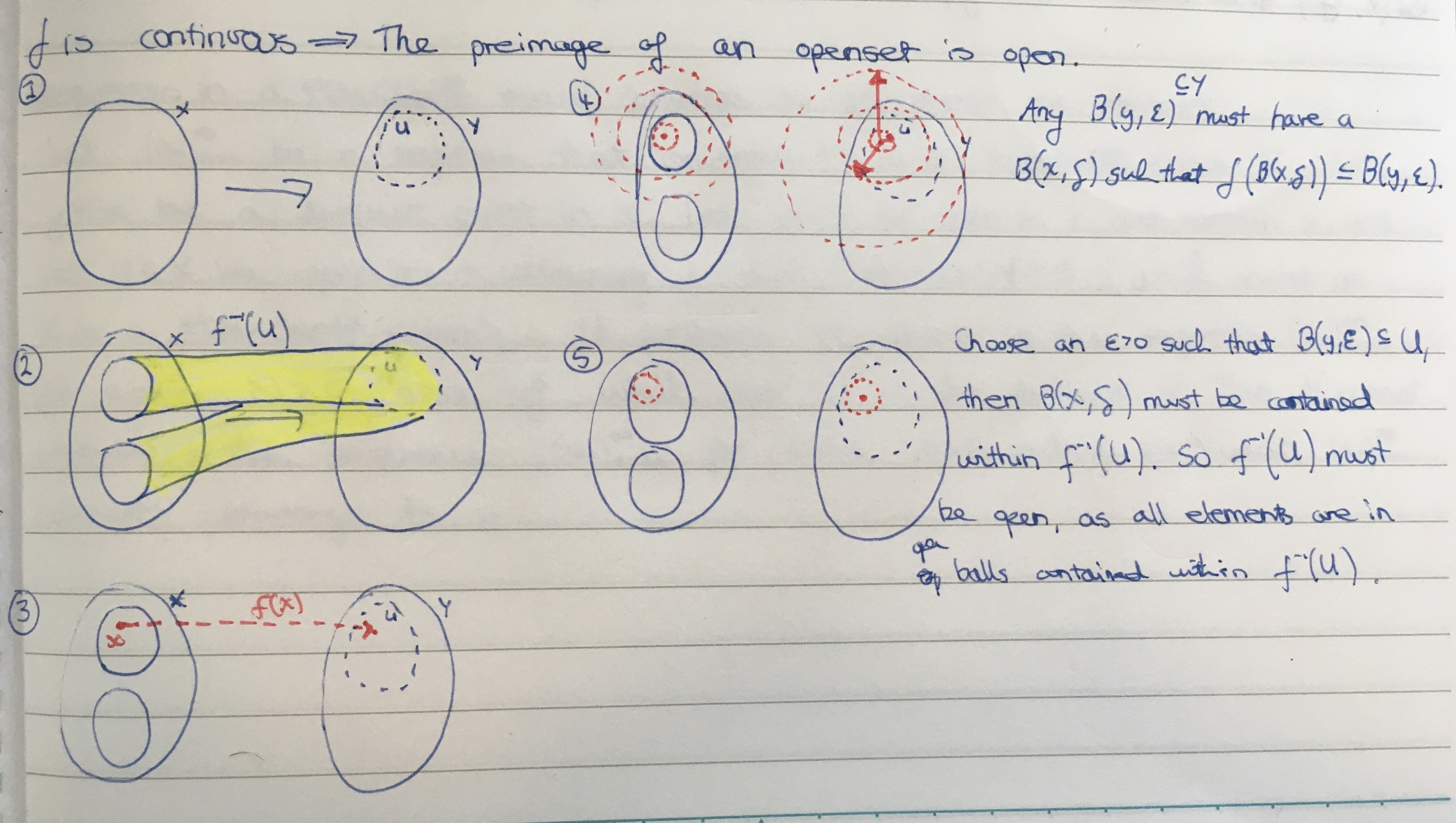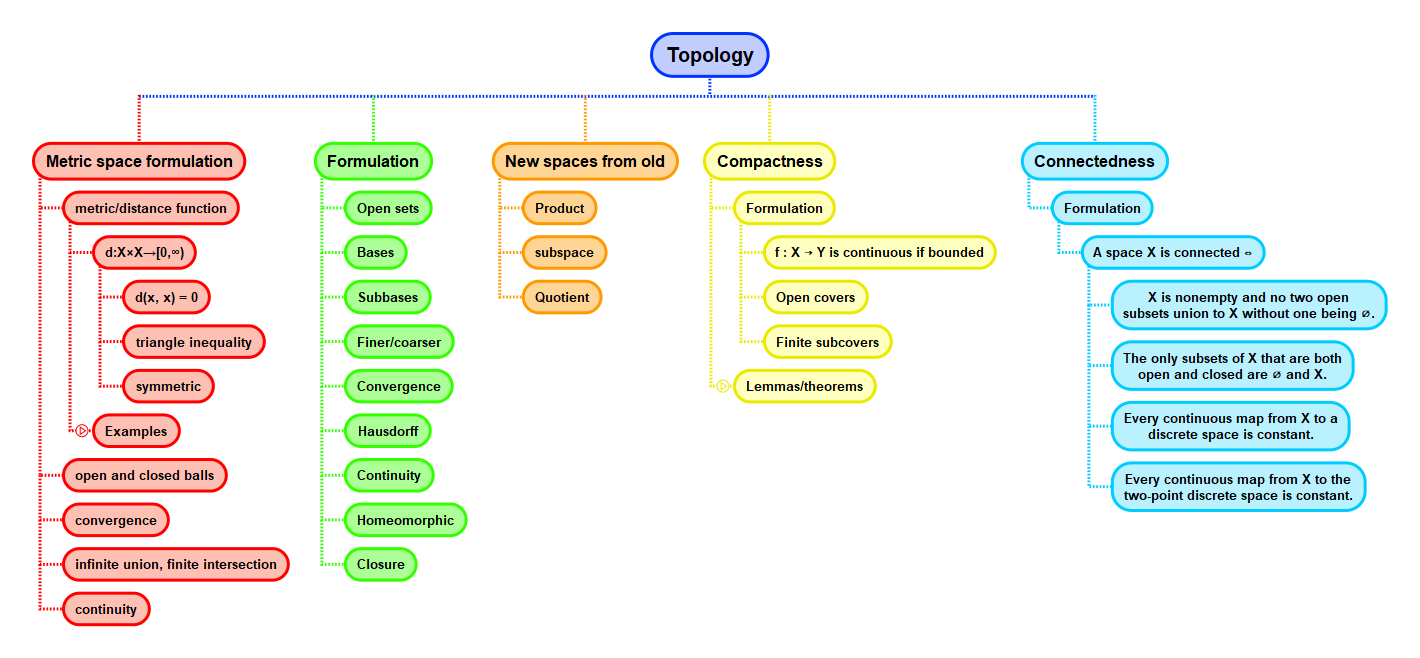Show Question
Math and science::Topology

# Metric space. Function continuity

### Function continuity between metric spaces

Let $$X$$ and $$Y$$ be metric spaces and let $$f: X \to Y$$ be a function. We say that $$f$$ is continuous iff:

For all $$x_0 \in X$$ and for all $$\varepsilon > 0$$ there exists a $$\delta > 0$$ such that ($$x \in B(x_0, \delta)$$ $$\implies$$ $$f(x) \in B(f(x_0), \varepsilon)$$).

### Independence of metrics

The idea of continuity appears to depend on the notion of metric/distance as it is formulated using ε-balls. However, the following lemma reveals that this is not really so—all that is needed is the notion of open (or closed) subsets.

#### Equivance to function continuity

Let $$X$$ and $$Y$$ be metric spaces and let $$f : X \to Y$$ be a function. The the following three statements are equivalent:

1. $$f$$ is continuous;
2. for all open $$U \subseteq Y$$, the preimage $$f^{-1}U \subseteq X$$ is open;
3. for all closed $$V \subseteq Y$$, the preimage $$f^{-1}V \subseteq X$$ is closed.

Informally: 'the preimage of an open set is open'.

Proof at the bottom of the back side.

This result motivates the definition of a topolgical space.

### The transition to Topology

With function continuity as the target concept, what is the minimum set of ideas requiring construction before function continuity can be formulated?

Without introducing the idea of a metric function, a space which we will call a topological space will be defined as being a set $$X$$ paired with a collection of subsets, to be called open subsets. These subsets will be defined to satisfy the 3 properties that were previously derived for metric spaces:

1. Arbitrary unions of open subsets are open.
2. Finite intersections of open subsets are open.
3. $$\emptyset$$ and $$X$$ are open.

This formulation could equally have been done with closed rather than open subsets.

After this restructuring, a function will be defined to be continuous iff 'the preimage of an open set is open'.

### Equivalence of Continuity, proof

Below is a proof for metric spaces of the equivalence of the three statements above regarding continuity. Below again is an attempt to visualize the line of reasoning.The main 'trick' of the proof is to iterate through each $$y \in Y$$. Consider the ε-ball around each $$y$$ that must be within $$U$$, and then, by the assumption of our definition of continuity applying to $$f$$, we can create an ε-ball around every $$x' = f^{-1}(y)$$. The the union of all these ε-balls in $$X$$ must be open.## Context